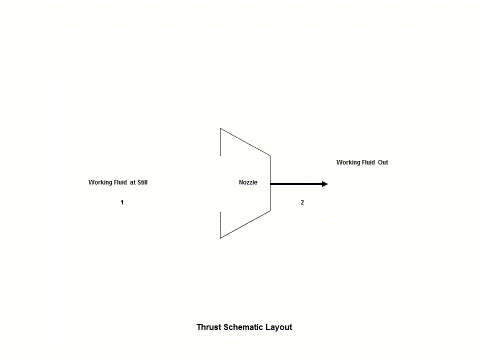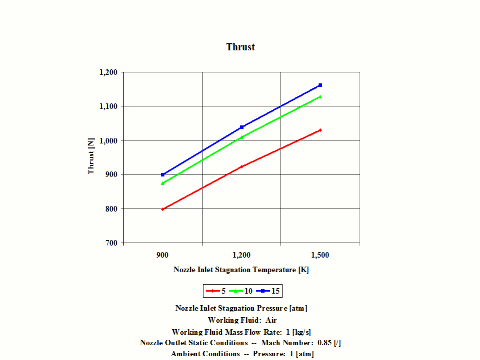top of page

### Thrust Analysis

Thrust

Introduction

This section provides an isentropic thrust analysis when the working fluid is air.

Analysis

In the presented thrust analysis, only air is considered as the working fluid behaving as a perfect gas -- specific heat has a constant value.  Ideal gas state equation is valid -- pv = RT.

Air enters a nozzle at point 1 and it exits the nozzle at point 2.  Isentropic expansion is considered with no entropy change.

Figure 1 contains a thrust schematic layout.Figure 1 - Thrust Schematic Layout

Figure 2 presents a thrust temperature vs entropy diagram.Figure 2 - Thrust Temperature vs Entropy Diagram

Figure 3 presents thrust performance as a function of the nozzle inlet stagnation temperature and pressure values for a few fixed values such as:  working fluid mass flow rate, nozzle outlet Mach Number and ambient pressure.  Only subsonic nozzle operation is considered.Figure 3 - Thrust Performance

One can notice that thrust increases with an increase in the inlet stagnation temperature and pressure values.

Assumptions

Working fluid is air.  There is no friction and heat transfer. Expansion is isentropic -- there is no entropy change.  Ideal gas state equation is valid -- pv = RT.  Air behaves as a perfect gas -- specific heat has a constant value.

Governing Equations

Tt/T = (1 + M^2(ϰ - 1)/2)
p
t/p = (1 + M^2(k - 1)/2)^
ϰ/(ϰ-1)
T
t/T = (pt/p)^(
ϰ-1)/ϰ
v = (2cp(Tt - T))^1/2
v
s = (
ϰRT)^1/2
M = v/v
s

ϰ = cp/cv
cp - cv = R
pv = RT
Thrust = vm + (p - p
a)A

Input Data

T1 = 900, 1,200 and 1,500 [K]
p
1 = 5, 10 and 15 [atm]
R = 0.2867 [kJ/kg*K]
c
p = 1.004 [kJ/kg*K]

ϰ = 1.4 [/]
m = 1 [kg/s]
M = 0.85 [/]
p
a = 1 [atm]

Results

Thrust Performance vs Nozzle Inlet Stagnation Temperature and Pressure
Outlet Mach Number = 0.85 [/] and Ambient Pressure = 1 [atm]
Working Fluid Mass Flow Rate = 1 [kg/s]

Thrust
[N]

Inlet Stagnation Temperature
[K]

Inlet Stagnation Pressure
[atm]
5

10

15

900

797.7

873.5

898.7

1,200

922.3

1,009.3

1,038.7

1,500

1,031.1

1,128.6

1,161.3

Conclusions

Thrust increases with an increase in the inlet stagnation temperature and pressure values.

References

JANAF Thermochemical Data - Tables, 1970

bottom of page